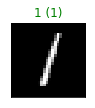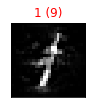# 2.5. Evasion Attacks against Neural Networks on MNIST dataset¶

Let’s continue from the Neural Networks tutorial, using the MNIST dataset this time. We can use a convolutional neural network, but we need to take care of reshaping the input to the expected input size, in this case (-1, 1, 28, 28). We will see in the following how to use torchvision’s transforms module for this purpose.

:

import torch
from torch import nn

class MNIST3cCNN(nn.Module):
"""Model with input size (-1, 28, 28) for MNIST 3-classes dataset."""
def __init__(self):
super(MNIST3cCNN, self).__init__()
self.conv1 = nn.Conv2d(1, 10, kernel_size=5)
self.conv2 = nn.Conv2d(10, 20, kernel_size=5)
self.conv2_drop = nn.Dropout2d()
self.fc1 = nn.Linear(320, 50)
self.fc2 = nn.Linear(50, 3)

def forward(self, x):
x = torch.relu(torch.max_pool2d(self.conv1(x), 2))
x = torch.relu(torch.max_pool2d(self.conv2_drop(self.conv2(x)), 2))
x = x.view(-1, 320)
x = torch.relu(self.fc1(x))
return self.fc2(x)


Now we can load MNIST dataset. Remember the input shape is (1, 1, 28, 28), using NCHW convention.

The input shape is an input parameter of the wrapper, since it has to take care of input reshaping before passing it to the neural network.

:

n_ts = 1000  # number of testing set samples

digits = (1, 5, 9)

# Normalize the data
tr.X /= 255
ts.X /= 255


Now we can use again the CClassifierPyTorch wrapper for having the model accessible with our library. Note that we pass the input shape as input parameter for the wrapper.

:

from torch import optim

# Random seed
torch.manual_seed(0)

net = MNIST3cCNN()
criterion = nn.CrossEntropyLoss()
optimizer = optim.SGD(net.parameters(),
lr=0.01, momentum=0.9)

from secml.ml.classifiers import CClassifierPyTorch
clf = CClassifierPyTorch(model=net,
loss=criterion,
optimizer=optimizer,
epochs=20,
batch_size=20,
input_shape=(1, 28, 28),
random_state=0)


To save time, we now load from the model zoo the pre-trained model.

:

# NBVAL_IGNORE_OUTPUT


And now we can check how well we can classify the digits.

:

label_torch = clf.predict(ts.X, return_decision_function=False)

from secml.ml.peval.metrics import CMetric
metric = CMetric.create('accuracy')
acc_torch = metric.performance_score(ts.Y, label_torch)

print("Model Accuracy: {}".format(acc_torch))

Model Accuracy: 0.997


## 2.5.1. Crafting Evasion Attacks¶

We can now create, as we did in notebook MNIST tutorial, adversarial examples against the neural network we just trained. The code is similar to the other notebook, the only difference will be the classifier that we pass to the CAttackEvasionPGDLS object.

:

# For simplicity, let's attack a subset of the test set
attack_ds = ts[:10, :]

noise_type = 'l2'  # Type of perturbation 'l1' or 'l2'
dmax = 3.0  # Maximum perturbation
lb, ub = 0., 1.  # Bounds of the attack space. Can be set to None for unbounded
y_target = None  # None if error-generic or a class label for error-specific

# Should be chosen depending on the optimization problem
solver_params = {
'eta': 0.5,
'eta_min': 2.0,
'eta_max': None,
'max_iter': 100,
'eps': 1e-6
}

pgd_ls_attack = CAttackEvasionPGDLS(classifier=clf,
surrogate_classifier=clf,
surrogate_data=tr,
distance=noise_type,
dmax=dmax,
solver_params=solver_params,
y_target=y_target)

print("Attack started...")
eva_y_pred, _, eva_adv_ds, _ = pgd_ls_attack.run(
attack_ds.X, attack_ds.Y, double_init=True)
print("Attack complete!")

Attack started...
Attack complete!

:

acc = metric.performance_score(
y_true=attack_ds.Y, y_pred=clf.predict(attack_ds.X))
acc_attack = metric.performance_score(
y_true=attack_ds.Y, y_pred=eva_y_pred)

print("Accuracy on reduced test set before attack: {:.2%}".format(acc))
print("Accuracy on reduced test set after attack: {:.2%}".format(acc_attack))

Accuracy on reduced test set before attack: 100.00%
Accuracy on reduced test set after attack: 10.00%


Finally, we can display the adversarial digit along with its label.

:

from secml.figure import CFigure
# Only required for visualization in notebooks
%matplotlib inline

# Let's define a convenience function to easily plot the MNIST dataset
def show_digits(samples, preds, labels, digs, n_display=8):
samples = samples.atleast_2d()
n_display = min(n_display, samples.shape)
fig = CFigure(width=n_display*2, height=3)
for idx in range(n_display):
fig.subplot(2, n_display, idx+1)
fig.sp.xticks([])
fig.sp.yticks([])
fig.sp.imshow(samples[idx, :].reshape((28, 28)), cmap='gray')
fig.sp.title("{} ({})".format(digits[labels[idx].item()], digs[preds[idx].item()]),
color=("green" if labels[idx].item()==preds[idx].item() else "red"))
fig.show()

show_digits(attack_ds.X[0, :], clf.predict(attack_ds.X[0, :]), attack_ds.Y[0, :], digits)